# Technical NotesCharacteristic Impedance The Characteristic Impedance of a coaxial transmission line is a function of the ratio of the inner and outer conductor diameters and the dielectric constant of the insulating medium.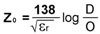Where: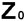- Characteristic Impedance D - Inside diameter of the outer conductor (inches) O - Diameter of the inner conductor (inches)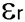- Dielectric constantVoltage Standing Wave Ratio (VSWR) VSWR is the ratio of the maximum and adjacent minimum wave, expressed in terms of reflection coefficient.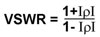VSWR is a real number; a value 1.0 implies a perfectly matched load.Insertion Loss

The composite Insertion Loss of a transmission line is determined by the loss associated with the inner and outer conductor, the dielectric medium and characteristic impedance mismatches.

1. Conductor Loss is a function of transmission line dimensions and materials.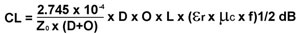Where: CL - Conductor Loss (dB) L - Conductor length (inches)- Characteristic Impedance (Ohms)- Dielectric constant D - Inside diameter of the outer conductor (inches)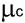- Dielectric constant O - Diameter of the inner conductor (inches) f - Frequency (Hz)

2. Dielectric Losses.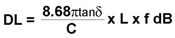Where: DL - Dielectric Loss (dB)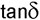- Loss tangent C - Velocity of propagation L - Conductor length (inches) f - Frequency (Hz)

3. Mismatch Loss is a function of reflected energy due to deviations from the characteristic impedance of the transmission line system.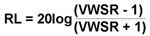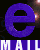Alpha Center 351 Irving Drive, Oxnard, CA 93030, USA ©2021 Alpha Products, Inc. All rights reserved.